# Zero Force from 3 charges placed at the vertices of a Triangle

cwill53
Homework Statement:
Two positive ions and one negative ion are fixed at the vertices of
an equilateral triangle. Where can a fourth ion be placed, along the
symmetry axis of the setup, so that the force on it will be zero? Is
there more than one such place? You will need to solve something
numerically.
Relevant Equations:
$$\vec{F}=\frac{1}{4\pi \varepsilon _0}\sum_{j=1}^{N}\frac{q_0q_j\hat{r}_{oj}}{r^{2}_{oj}}$$
I'm not really sure where to start with this problem, but I wanted to ask a few questions about the approach I should use.

Is it reasonable to say that a gradient could be set up that could describe the force on the fourth ion at any point?

The way I'm thinking of this problem is, I want to find how the sum of the forces on the fourth ion change as the fourth ion moves through space.
But I'm not sure if this is the correct way to think about it or how to solve this mathematically.

Last edited:

Homework Helper
Gold Member
2022 Award
Is it reasonable to say that a gradient could be set up that could describe the force on the fourth ion at any point?
Yes, the potential gradient, which would be the net field. You don't know and don't care what the actual force would be since that depends on the magnitude of the test charge.

•cwill53
cwill53
Yes, the potential gradient, which would be the net field. You don't know and don't care what the actual force would be since that depends on the magnitude of the test charge.

I still don't really understand how I should go about solving.

cwill53
Yes, the potential gradient, which would be the net field. You don't know and don't care what the actual force would be since that depends on the magnitude of the test charge.

I saw a post of yours from a few days ago, saying:

"I don't see how the polarity of the test ion matters. A zero field is a zero field.

Assuming all three charges have the same magnitude, there will will always be a saddle point in the potential near the midpoint between the two like charges, just a little further away than that from the third charge, and another on the far side of that third charge, roughly twice as far from the like charges as from the third charge.

For each of the combinations of of one charge and one saddle point, there is a unique field line connecting them. Using the diagram layout, there is a field line from the lower left charge to each saddle point, and a straight field line from each of those to the top charge. Together, these lines bound roughly a semicircle. Inside that, all field lines run from the lower left charge to the top charge. This leaves no possibility of another saddle point in that region.
Etc."

Homework Helper
Gold Member
2022 Award
Consider a point on the symmetry axis at distance x from the midpoint of the two like charges (I think that will be simplest).
Write vector expressions for the fields the three charges exert at that point. You can omit the opposing components from the two like charges as these will cancel.
What do you get?

•cwill53
cwill53
Consider a point on the symmetry axis at distance x from the midpoint of the two like charges (I think that will be simplest).
Write vector expressions for the fields the three charges exert at that point. You can omit the opposing components from the two like charges as these will cancel.
What do you get?
First I'll show you the picture I drew so you can follow the steps I took and make corrections along the way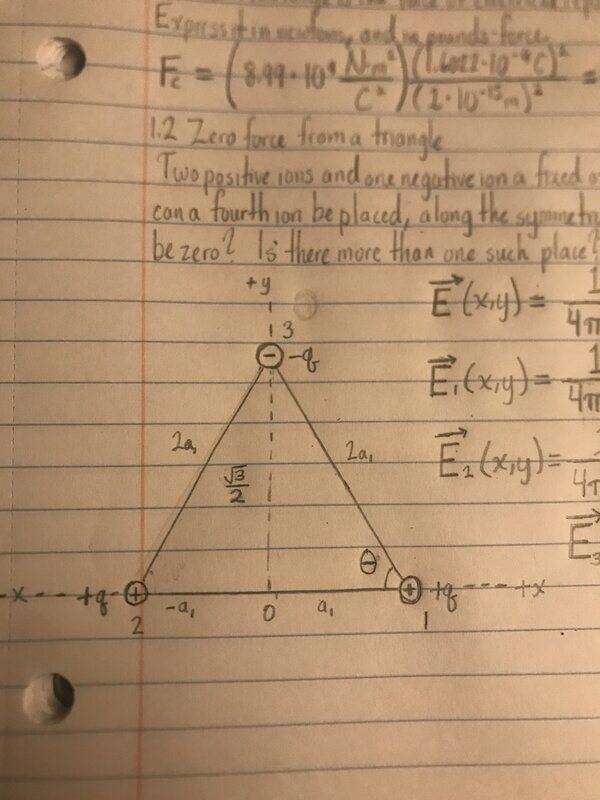What I wrote was:

$$\vec{E}(x,y)=\frac{1}{4\pi \varepsilon _0}\sum_{j=1}^{N}\frac{q_j\hat{r}_{oj}}{r^{2}_{oj}}$$

$$\vec{E}_1(x,y)=\frac{1}{4\pi \varepsilon _0} \frac{q_1\left \langle -a_1,2a_1cos\theta \right \rangle}{(\sqrt{(a_1)^2+(2a_1cos\theta )^2})^2}$$

$$\vec{E}_2(x,y)=\frac{1}{4\pi \varepsilon _0} \frac{q_2\left \langle a_1,2a_1cos\theta \right \rangle}{(\sqrt{(a_1)^2+(2a_1cos\theta )^2})^2}$$

$$\vec{E}_3(x,y)=\frac{1}{4\pi \varepsilon _0} \frac{-q_3\left \langle 0,\frac{\sqrt{3}}{2}-2a_1cos\theta \right \rangle}{(\sqrt{(\frac{\sqrt{3}}{2}-2a_1cos\theta )^2})^2}$$

Where ##2a_1cos \theta=y##.

Homework Helper
Gold Member
2022 Award
$$\vec{E}(x,y)=\frac{1}{4\pi \varepsilon _0}\sum_{j=1}^{N}\frac{q_j\hat{r}_{oj}}{r^{2}_{oj}}$$

$$\vec{E}_1(x,y)=\frac{1}{4\pi \varepsilon _0} \frac{q_1\left \langle -a_1,2a_1cos\theta \right \rangle}{(\sqrt{(a_1)^2+(2a_1cos\theta )^2})^2}$$
You can simplify the algebra by just taking the triangle to have side length 2.

The generic equation is right , but note the numerator has ##\hat r##. In your later equations you have treated it as ##\vec r##.

I don't understand those later equations though. On the left you have the field as a function of x and y, but neither appears in the right. At what point do you think you are calculating the fields?
Anyway, you don't care about the x component. At points on the y-axis those will cancel.
Pick a point at (0,y) and write the expressions for the three ##E_y## fields as scalars.

•cwill53
cwill53
You can simplify the algebra by just taking the triangle to have side length 2.

The generic equation is right , but note the numerator has ##\hat r##. In your later equations you have treated it as ##\vec r##.

I don't understand those later equations though. On the left you have the field as a function of x and y, but neither appears in the right. At what point do you think you are calculating the fields?
Anyway, you don't care about the x component. At points on the y-axis those will cancel.
Pick a point at (0,y) and write the expressions for the three ##E_y## fields as scalars.
Hmmmm, is it true that y is equal to ##2a_1cos\theta##? That's what I was trying to do there, I just should've replaced that with y.

I had copied that equation straight from Purcell's book, which so far has a few notation errors. I think ##\hat r## should really be ##\vec r## because the explanation in the book says ##\vec{r}_{0j}## is the vector from the j-th charge in the system to the point (x,y,z).

The fields are supposed to be calculated on the y axis, and since the x components of ##\vec{E}_1## and ##\vec{E}_2## are opposite, they cancel.

Homework Helper
Gold Member
2022 Award
Hmmmm, is it true that y is equal to 2a1cosθ?
y is the coordinate of a point you are trying to find. Looks like you are calculating the field at one ion due to the charge at another. That isn't useful at all.
I think r^ should really be r→
No. The field at ##\vec r## from a charge q is
$$\frac{1}{4\pi \varepsilon _0}\frac{q\hat{r}}{r^{2}}$$
which you can also write as
$$\frac{1}{4\pi \varepsilon _0}\frac{q\vec{r}}{r^{3}}$$
Note the two differences.
It is not $$\frac{1}{4\pi \varepsilon _0}\frac{q\vec{r}}{r^{2}}$$

•cwill53
cwill53
y is the coordinate of a point you are trying to find. Looks like you are calculating the field at one ion due to the charge at another. That isn't useful at all.

No. The field at ##\vec r## from a charge q is
$$\frac{1}{4\pi \varepsilon _0}\frac{q\hat{r}}{r^{2}}$$
which you can also write as
$$\frac{1}{4\pi \varepsilon _0}\frac{q\vec{r}}{r^{3}}$$
Note the two differences.
It is not $$\frac{1}{4\pi \varepsilon _0}\frac{q\vec{r}}{r^{2}}$$
What I should be trying to figure out is the y-coordinate where the field is zero, because if the field is zero, the force on any charge regardless of its sign would be zero, right?

Aside from the fact that the magnitudes of the distances in the denominators of each of equations should be cubed instead of squared, I'm trying to figure out how this approach wouldn't work.

What I want to know is the field at a point (0,y) due to an arrangement of charges.

Homework Helper
Gold Member
2022 Award
What I want to know is the field at a point (0,y) due to an arrangement of charges.
Then why doesn't the variable y appear in any of your field expressions?
In your generic equation, the scalar r is the distance from an ion to your test charge at (0,y). So for the ion at lower right r is the distance from (0,y) to (a,0).

•cwill53
cwill53
Then why doesn't the variable y appear in any of your field expressions?
In your generic equation, the scalar r is the distance from an ion to your test charge at (0,y). So for the ion at lower right r is the distance from (0,y) to (a,0).
I realized that for one the cosine should be sine in all of my equations, is this correct? I was also told that my equations don't give the field for an arbitrary y.
$$\vec{E}(x,y)=\frac{1}{4\pi \varepsilon _0}\sum_{j=1}^{N}\frac{q_j\hat{r}_{oj}}{r^{2}_{oj}}=\frac{1}{4\pi \varepsilon _0}\sum_{j=1}^{N}\frac{q_j\vec{r}_{oj}}{r^{2}_{oj}}\frac{1}{\left \|\vec{r}_{oj} \right \|}$$
$$\vec{E}_1(x,y)=\frac{1}{4\pi \varepsilon _0} \frac{q_1\left \langle -a_1,y \right \rangle}{(\sqrt{(a_1)^2+(y)^2})^3}$$
$$\vec{E}_2(x,y)=\frac{1}{4\pi \varepsilon _0} \frac{q_2\left \langle a_1,y \right \rangle}{(\sqrt{(a_1)^2+(y )^2})^3}$$
$$\vec{E}_3(x,y)=\frac{1}{4\pi \varepsilon _0} \frac{-q_3\left \langle 0,2a_1sin\theta-y \right \rangle}{(\sqrt{(2a_1sin\theta -y )^2})^3}$$

Do these equations make more sense?

Homework Helper
Gold Member
2022 Award
I realized that for one the cosine should be sine in all of my equations, is this correct? I was also told that my equations don't give the field for an arbitrary y.
$$\vec{E}(x,y)=\frac{1}{4\pi \varepsilon _0}\sum_{j=1}^{N}\frac{q_j\hat{r}_{oj}}{r^{2}_{oj}}=\frac{1}{4\pi \varepsilon _0}\sum_{j=1}^{N}\frac{q_j\vec{r}_{oj}}{r^{2}_{oj}}\frac{1}{\left \|\vec{r}_{oj} \right \|}$$
$$\vec{E}_1(x,y)=\frac{1}{4\pi \varepsilon _0} \frac{q_1\left \langle -a_1,y \right \rangle}{(\sqrt{(a_1)^2+(y)^2})^3}$$
$$\vec{E}_2(x,y)=\frac{1}{4\pi \varepsilon _0} \frac{q_2\left \langle a_1,y \right \rangle}{(\sqrt{(a_1)^2+(y )^2})^3}$$
$$\vec{E}_3(x,y)=\frac{1}{4\pi \varepsilon _0} \frac{-q_3\left \langle 0,2a_1sin\theta-y \right \rangle}{(\sqrt{(2a_1sin\theta -y )^2})^3}$$

Do these equations make more sense?
Yes, much better. But the E(x,y) should be E(0,y).
Next step is to add up the y components of the fields, since the x components clearly cancel.

•cwill53
cwill53
Yes, much better. But the E(x,y) should be E(0,y).
Next step is to add up the y components of the fields, since the x components clearly cancel.
Should I assume that the magnitude of each ion's charge is the same?

Well, obviously I should if the components cancel out.

Homework Helper
Gold Member
2022 Award
Should I assume that the magnitude of each ion's charge is the same?

Well, obviously I should if the components cancel out.
The cancellation only depends on the two like charges having the same magnitude.
But since you are told you'll have to use a numerical approximation you would need to know the relative magnitudes of all the charges, so I would assume the three are equal.

•cwill53
cwill53
The cancellation only depends on the two like charges having the same magnitude.
But since you are told you'll have to use a numerical approximation you would need to know the relative magnitudes of all the charges, so I would assume the three are equal.
After taking your advice to simplify the algebra some, I set up the values such that ##q_1=q_2=1C## , ##q_3=-1C##, ##a_1=1m##, and ##k_e=8.99\cdot 10^9\frac{N\cdot m^2}{C^2}##.
Doing that and removing the units, I got the equation

$$\:\left(8.99\cdot \:10^9\right)\left(\frac{2y}{\left(1+y^2\right)^{\frac{3}{2}}}+\frac{-2sin\left(60^{\circ }\right)+y}{\left(2sin\left(60^{\circ }\right)-y\right)^3}\right)=0$$

I put this into Wolfram Alpha's solver and got the following: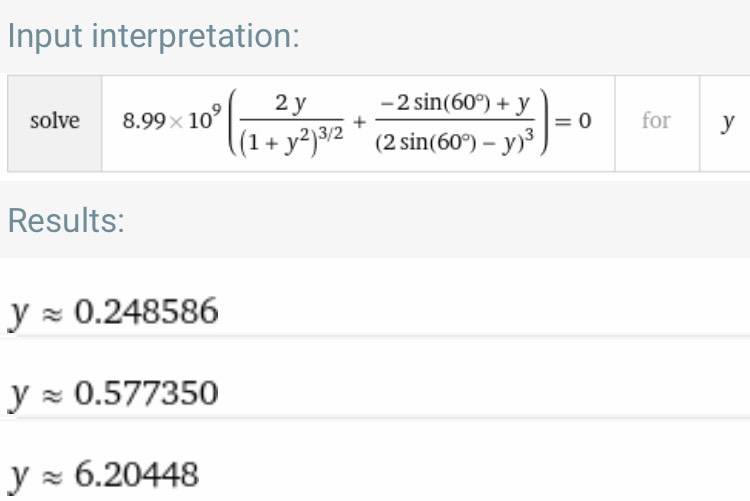However, I looked on Slader to see if someone else got this solution, and I saw this:

I'm not sure who's right. We got one shared value.

Last edited:
Homework Helper
Gold Member
2022 Award
After taking your advice to simplify the algebra some, I set up the values such that ##q_1=q_2=1C## , ##q_3=-1C##, ##a_1=1m##, and ##k_e=8.99\cdot 10^9\frac{N\cdot m^2}{C^2}##.
Doing that and removing the units, I got the equation

$$\:\left(8.99\cdot \:10^9\right)\left(\frac{2y}{\left(1+y^2\right)^{\frac{3}{2}}}+\frac{-2sin\left(60^{\circ }\right)+y}{\left(2sin\left(60^{\circ }\right)-y\right)^3}\right)=0$$

I put this into Wolfram Alpha's solver and got the following:
View attachment 268902

However, I looked on Slader to see if someone else got this solution, and I saw this:

I'm not sure who's right. We got one shared value.
You have the power 3/2 in one denominator but you forgot the /2 in the other.
Edit: scratch that.. just realized you removed a power of 2,

Also, just occurred to me that you need to take the absolute values in the denominators.
##4y^2(y-\sqrt 3)^6=(y-\sqrt 3)^2(1+y^2)^3##.

You don't need the constant factor out the front.

Last edited:
cwill53
You have the power 3/2 in one denominator but you forgot the /2 in the other.
Also, just occurred to me that you need to take the absolute values in the denominators.

You don't need the constant factor out the front.
Shouldn't the root in the following denominator cancel due to the the square term?

$$\vec{E}_3(x,y)=\frac{1}{4\pi \varepsilon _0} \frac{-q_3\left \langle 0,2a_1sin\theta-y \right \rangle}{(\sqrt{(2a_1sin\theta -y )^2})^3}$$

Homework Helper
Gold Member
2022 Award
Shouldn't the root in the following denominator cancel due to the the square term?

$$\vec{E}_3(x,y)=\frac{1}{4\pi \varepsilon _0} \frac{-q_3\left \langle 0,2a_1sin\theta-y \right \rangle}{(\sqrt{(2a_1sin\theta -y )^2})^3}$$
Yes, I was in the process of correcting my post. See update.

•cwill53
cwill53
You have the power 3/2 in one denominator but you forgot the /2 in the other.
Edit: scratch that.. just realized you removed a power of 2,

Also, just occurred to me that you need to take the absolute values in the denominators.
##4y^2(y-\sqrt 3)^6=(y-\sqrt 3)^2(1+y^2)^3##.

You don't need the constant factor out the front.

So should I take the absolute value of the denominators in this equation? I expanded the expressions out and put ##\frac{\sqrt{3}}{2}## for sin(60):
$$\frac{2y}{\sqrt{1+y^2}(1+y^2)}+\frac{-\sqrt{3}+y}{\frac{(\sqrt{3}-2y)^3}{8}}$$

Homework Helper
Gold Member
2022 Award
So should I take the absolute value of the denominators in this equation?
Yes.

Homework Helper
Gold Member
I think your expressions are not completely correct. One thought I had is that you start over, and write the distance to the center one in much simpler form, and simply write the cosines for the other two charges in terms of ##y ##. You should be able to write a single expression that then needs to be solved for ## y ##, or actually more precisely as a function of ## u=y/h ##, where ## h ## is the altitude of the triangle. Perhaps @haruspex might have a different opinion, but that is my input.

•cwill53
cwill53
Yes.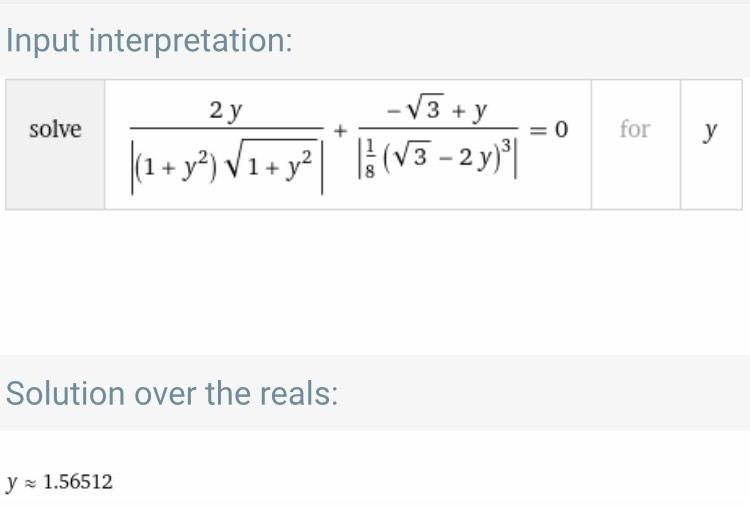I tried this, and the solutions I got were wrong.

Homework Helper
Gold Member
One thing that is confusing is where the origin is for the distance ## y ##. The OP might be using the base of the triangle=in my post 22, perhaps that is why my expressions are different.
Edit: In the latest post, I think the OP needs to be consistent with his triangle=if you use ## 1 ## for the distance of half the base, then the altitude is ## \sqrt{3} ##.

Last edited:
•cwill53
cwill53
One thing that is confusing is where the origin is for the distance ## y ##. The OP might be using the base of the triangle=in my post 22, perhaps that is why my expressions are different.
I was using the base of the triangle as the origin.

In my original equations, I had got one of the points correct when I put it in Wolfram Alpha. I just don't see why I didn't get the other correct point.

•Homework Helper
Gold Member
Please see the Edit to post 24.

•cwill53
Homework Helper
Gold Member
The other correct point comes from a different scenario. If you go slightly below the base, that is where you will find the other point. You will new a new set of equations to get that point.

•cwill53
Homework Helper
Gold Member
In your post 16, I think I agree with the answer of 6.20. In that post, you are using ## \sqrt{3} ## for the altitude of the triangle. Your formulas are consistent there. In post 23, you didn't maintain consistency.

•cwill53
cwill53
Please see the Edit to post 24.
I can't see where I was inconsistent with the triangle.

If the triangle is equilateral, and I set half the length of it equal to 1, then the length of the y-axis is 2(1)sin(60). This is the square root of 3.

I'm starting to get really confused now. The solutions to the following equation:

$$(\frac{2y}{(1+y^{2})^{\frac{3}{2}}}+\frac{-2sin(60^{\circ})+y}{(2sin(60^{\circ})-y)^{3}})=0$$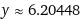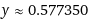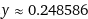It occurred to me to just play around with it, and I got the following solution to the following equation:
$$-\frac{2y}{\left(1+y^2\right)^{\frac{3}{2}}}=\frac{-2sin\left(60\right)+y}{\left(y-2sin\left(60\right)\right)^3}$$
y≈−0.14629…

This value is the negative value of what the guy in Slader got. Is this a coincidence?

Homework Helper
Gold Member
The top part of 29 is good. I agree with 6.20 as the answer. The other solutions are meaningless. To get the other point, it is going to be slightly below the base. You are going to need to write new expressions with the new scenario to find this other point.

•cwill53
cwill53
The top part of 29 is good. I agree with 6.20. The other solutions are meaningless. To get the other point, it is going to be slightly below the base. You are going to need to write new expressions with the new scenario to find this other point.
Did you see the other solution when I switched the terms around in the denominator of the right hand side term in post #29? Does this make sense?

Homework Helper
Gold Member
Did you see the other solution when I switched the terms around in the denominator of the right hand side term in post #29?
It could be a mathematical coincidence. The answer might agree with the right answer, but you need to work through the calculation to find the second point. You should be able to see qualitatively before you compute the exact location, why the second point is just slightly below the base.

•cwill53
cwill53
It could be a mathematical coincidence. The answer might agree with the right answer, but you need to work through the calculation to find the second point. You should be able to see qualitatively before you compute the exact location, why the second point is just slightly below the base.
Alright.

I'm not too sure what new expressions I should write, or how I should approach it. I thought that the expressions I created would give me the field for an arbitrary point in space.

Homework Helper
Gold Member
Suggestion for you on this one (the second point) to make it easier: Call the distance below the base positive ## y ##. The distance from the negative charge is then ## y+\sqrt{3} ##. With the practice you had from the first part, it should be simple matter to write ## \frac{1}{(y+\sqrt{3})^2}=2\frac{y}{(...)^{3/2}} ##.

•cwill53
•